# I.E. Irodov Solutions on Elastic Deformations Of A Solid Body

JEE aspirants can find the solutions of the Problems In General Physics I.E. Irodov - Elastic Deformations Of A Solid Body on this page. This is an important topic in Physical Fundamentals of Mechanics. The branch of physics which deals with the motion and the forces responsible for it is called mechanics. The main topics in Elastic Deformations Of A Solid Body are the relation between stress and strain, compressibility, relation between shear strain and tangential stress, volume density of elastic strain- energy, etc.

Revising these solutions will help students to achieve better results in the JEE exam and other competitive exams.

### I.E. Irodov Solutions on Dynamics OF A Solid Body

1. What pressure has to be applied to the ends of a steel cylinder to keep its length constant on raising its temperature by 1000C?

Solution:

1. Variation of length with temperature is given by

lt = l0(1+α∆t) or ∆l/l0 = α∆t = ε (1)

But ε = σ/E,

Thus σ = α∆tE, which is the sought stress of pressure.

Putting the value of α and E from Appendix and taking ∆t = 1000C, we get

σ = 2.2×103 atm.

2. What internal pressure (in the absence of an external pressure ) can be sustained

1. (a) by a glass tube;

2. (b) by a glass spherical flask, if in both cases the wall thickness is equal tor = 1.0 mm and the radius of the tube and the flask equals r = 25 mm?

Solution:

1. (a) Consider a transverse section of the tube and concentrate on an element which subtends an angle ∆ϕ at the centre. The forces acting on a portion of length ∆l on the element are

(1) tensile forces sideways of magnitude σ∆r∆l.

The resultant of these is

2σ∆r∆l sin ∆ϕ/2 ≈ σ∆r∆l ∆ϕ radially towards the centre.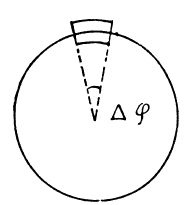(2) the force due to fluid pressure = pr∆ϕ∆l since these balance,

we get pmax ≈ σm∆r/r where σm is the maximum tensile force.

Putting the values we get pmax = 19. 7 atmos.

(b) Consider an element of area dS = π(r ∆θ/2)2 about z-axis chosen arbitrarily. There are tangential tensile forces all around the ring of the cap.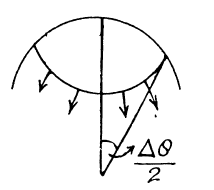Their resultant is

σ(2π(r∆θ/2)∆r sin ∆θ/2

Hence in the limit

pmπ(r∆θ/2)2 = σmπ(r∆θ/2)∆r ∆θ

Or pm = 2σm∆r/r = 39.5 atmos.

3. A horizontally oriented copper rod of length l = 1.0 m is rotated about a vertical axis passing through its middle. What is the number of rps at which this rod ruptures?

Solution:

1. Let us consider an element of the rod at a distance x from its rotation axis (Fig.).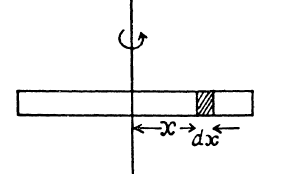From Newton’s second law in projection form directed towards the rotation axis

-dT = (dm)ω2 x = (m/l)ω2x dx

On integrating

-T = (mω2/l)x2/2 + C (constant)

But at x = ±l/2 or free end, T = 0

Thus 0 = (mω2/2)l2/4 + C or C = -mω2l/8

Hence T = (mω2/2)(l/4 - x2/l)

Thus Tmax = mω2l/8 (at midpoint)

Condition required for the problem is

Tmax = Sσm

So mω2l/8 = Sσm or ω = (2/l)√(2σm/ρ)

Hence the sought number of rps is

n = ω/2π

= (1/πl)√(2σm/ρ) (using the table n = 0.8×102 rps)

4. A ring of radius r = 25 cm made of lead wire is rotated about a stationary vertical axis passing through its centre and perpendicular to the plane of the ring. What is the number of rps at which the ring ruptures?

Solution:

1. Let us consider an element of the ring (Fig.).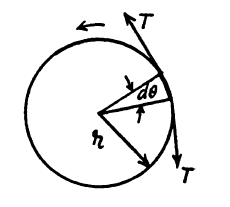From Newton’s law Fn = mwn for this element, we get,

Tdθ = (m/2π)dθ ω2r

So T = (m/2π)ω2r

Condition for the problem is

T/πr2 ≤ σm or, mω2r/2π2r2 ≤ σm

Or ω2max = 2ω2σmr/πr2(2πrρ) = σm/ρr2

Thus sought number of rps

n = ωmax/2π

= (1/2πr)√(σm/ρ)

Using the table of appendices n = 23 rps.

5. A steel wire of diameter d = 1.0 mm is stretched horizontally between two clamps located at the distance l = 2.0 m from each other. A weight of mass m = 0.25 kg is suspended from the midpoint O of the wire. What will the resulting descent of the point O be in centimetres?

Solution:

1. Let the point O descend by the distance x (Fig.).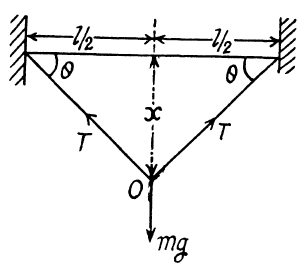From the condition of equilibrium of point O.

2T sin θ = mg

Or T = mg/2sin θ = (mg/2x)√((l/2)2 + x2) ..(1)

Now T/π(d/2)2 = σ = εE or T = εEπd2/4 ..(2)

(σ here is stress and ε is strain.)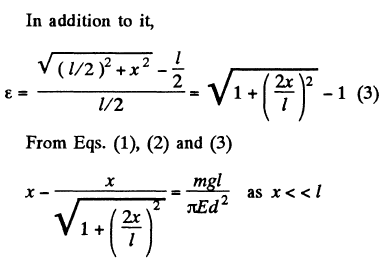So, 4x3/2l2 ≈ mgl/πEd2

Or x = l(mg/2πEd2) = 2.5 cm.

6. A uniform elastic plank moves over a smooth horizontal plane due to a constant force F0 distributed uniformly over the end face. The surface of the end face is equal to S, and Young's modulus of the material to E. Find the compressive strain of the plank in the direction of the acting force.

Solution:

1. Let us consider an element of the rod at a distance (Fig.).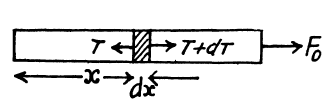For the considered element, ‘T - T’ are internal restoring forces which produce elongation and dT provides the acceleration to the element. For the element from Newton’s law :

dT = (dm)w

= (m/l)dx F0/m = (F0/l)dx

As free end has zero tension, on integrating the above expression,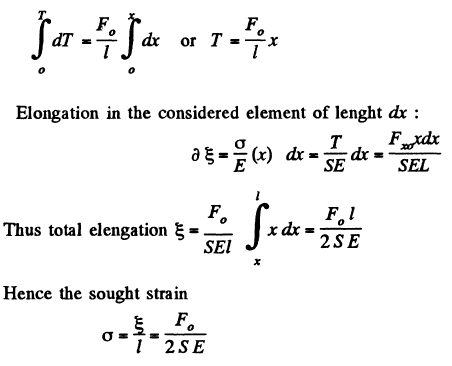7. A thin uniform copper rod of length 1 and mass m rotates uniformly with an angular velocity w in a horizontal plane about a vertical axis passing through one of its ends. Determine the tension in the rod as a function of the distance r from the rotation axis. Find the elongation of the rod.

Solution:

1. Let us consider an element of the rod at a distance r from its rotation axis. As the element rotates in a horizontal circle of radius r, we have from Newton’s second law in projection form directed toward the axis of rotation.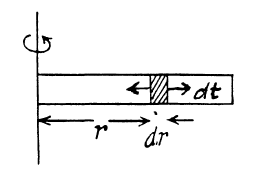T - (T + dT) = (dm)ω2r

-dT = (m/l)dr ω2r

= (m/l)ω2r dr

At the free end tension becomes zero. Integrating the above expression we get, thus

$-\int_{T}^{0}dT=\frac{m}{l}\omega ^{2}\int_{r}^{l}r\: dr$

Thus T = (mω2/l)(l2 - r2)/2

= (mω2l/2)(1 - r2/l2)

Elongation in elemental length dr is given by:

∂ξ = (σr/E)dr = (T/SE)dr

Where S is the cross sectional area of the rod and T is the tension in the rod at the considered element)

Or ∂ξ = (mω2l/2SE)(1 - r2/l2)dr

Thus the sought elongation

ξ = ∫dξ = (mω2l/2SE) ∫0l(1- r2/l2)dr

or , ξ = (mω2l/2SE)(2l/3)

= (Slρ/3SE)ω2l3

= ⅓ lρω2l3/E(where ρ is the density of the copper.)

8. A solid copper cylinder of length l = 65 cm is placed on a horizontal surface and subjected to a vertical compressive force F = 1000 N directed downward and distributed uniformly over the end face. What will be the resulting change of the volume of the cylinder in cubic millimetres?

Solution:

1. Volume of a solid cylinder, V = πr2l

So ∆V/V = π2r∆rl/πr2l + πr2∆rl/πr2l

= 2∆r/r + ∆l/l (1)

But longitudinal strain ∆l/l and accompanying lateral strain ∆r/r are related as

∆r/r = -μ ∆l/l (2)

Using (2) in (1), we get

∆V/V = (∆l/l) (1 - 2μ) (3)

∆l/l = -F/π r2/E

Because the increment in the length of cylinder ∆l is negative.

So, ∆V/V = (-F/π r2E)(1 - 2μ)

Thus, ∆V = (-Fl/E)(1 - 2μ)

Negative sign means that the volume of the cylinder has decreased.

9. A copper rod of length l is suspended from the ceiling by one of its ends. Find:

1. (a) the elongation Δl of the rod due to its own weight;

2. (b) the relative increment of its volume ΔVIV.

Solution:

1. (a) As free end has zero tension, thus the tension in the rod at a vertical distance y from its lower end

T = (m/l)gy ..(1)

Let ∂l be the elongation of the element of length dy, then

∂l = (σ(y)/E) dy

= (T/SE)dy

= mgy dy/SlE

= ρ gy dy/E (where ρ is the density of the copper)

Thus the sought elongation ∆l = ∫∂l = ρg∫0l y dy/E

= ½ ρgl2/E ..(2)

(b) If the longitudinal (tensile) strain is ε = ∆l/l, the accompanying lateral (compressive) strain is given by ε’ = ∆r/r = -με (3)

Then since V = πr2l

we have ∆V/V = 2∆r/r + ∆l/l

= (1 - 2μ)∆l/l (using (3))

Where ∆l/l is given in part (a), μ is the Poisson ratio for copper.

10. A bar made of material whose Young's modulus is equal to E and Poisson's ratio to μ, is subjected to the hydrostatic pressure P. Find:

1. (a) the fractional decrement of its volume;

2. (b) the relationship between the compressibility β and the elastic constants E and μ.

Solution:

1. Consider a cube of unit length before pressure is applied. The pressure acts on each face. The pressures on the opposite faces constitute a tensile stress producing longitudinal compression and lateral extension. The compression is P/E and the lateral extension is μP/E.

The net results is a compression.

P(1 - 2μ)/E in each side.

Hence ∆V/V = (-3P/E)(1 - 2μ) because from symmetry ∆V/V = 3∆l/l.

(b) Let us consider a cube under an equal compressive stress σ, acting on all its faces.

Then, volume strain = -∆V/V = σ/k

Where k is the bulk modulus of elasticity.

So σ/k = (3σ/E)(1 - 2μ)

Or E = 3k(1 - 2μ)

= (3/β)(1 - 2μ) (as k = 1/β)

μ ≤ ½ if E and β are both to remain positive.

11. One end of a steel rectangular girder is embedded into a wall (Fig. 1.74). Due to gravity it sags slightly. Find the radius of curvature of the neutral layer (see the dotted line in the figure) in the vicinity of the point O if the length of the protruding section of the girder is equal to l = 6.0 m and the thickness of the girder equals h = 10 cm.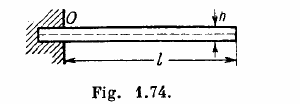Solution:

1. A beam clamped at one end and supporting an applied load at the free end is called a cantilever. The theory of cantilevers is discussed in advanced text book on mechanics. The key result is that elastic forces in the beam generate a couple, whose moment, called the moment of resistances, balances the external bending moment due to weight of the beam, load etc. The moment of resistance, also called internal bending moment (I.B.M) is given by

I.B.M. = EI/R

Here R is the radius of curvature of the beam at the representative point (x, y). I is called the geometrical moment of inertia

I = ∫z2 dS

of the cross section relative to the axis passing through the neutral layer which remains unstretched. (Fig.l.). The section of the beam beyond P exerts the bending moment N (x) and we have,

EI/R = N(x)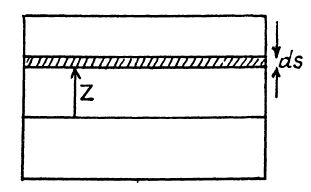If there is no load other than that due to the weight of the beam, then

N(x) = ½ ρg(l - x)2bh

Where ρ = density of steel.

Hence at x = 0,

(I/R)0 = ρgl2bh/2EI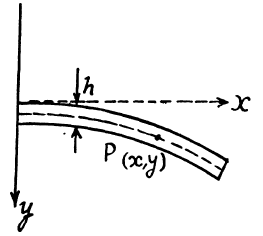Here b = width of the beam perpendicular to paper.

Also I = $\int_{\frac{-h}{2}}^{\frac{h}{2}}z^{2}\: bdz$

= bh3/12

Hence, (1/R)0 = 6ρgl2/Eh2 = (0.121 km)-1.

12. The bending of an elastic rod is described by the elastic curve passing through centres of gravity of rod’s cross-sections. At small bendings the equation of this curve takes the form N(x) = EI d2y/dx2 , where N (x) is the bending moment of the elastic forces in the cross section corresponding to the x coordinate, E is Young's modulus, I is the moment of inertia of the cross-section relative to the axis passing through the neutral layer (I = ∫z2dS, Fig. 1.75).

Suppose one end of a steel rod of a square cross-section with side a is embedded into a wall, the protruding section being of length l (Fig. 1.76). Assuming the mass of the rod to be negligible, find the shape of the elastic curve and the deflection of the rod λ, if its end A experiences

1. (a) the bending moment of the couple N0;

2. (b) a force F oriented along the y axis.

Solution:

1. We use the equation given above and use the result that when y is small

1/R ≈ d2y/dx2

Thus d2y/dx2 = N(x)/EI

(a) here N(x) = N0 is a constant. Then integration gives,

dy/dx = N0x/EI + C1

But dy/dx = 0 for x = 0, so C1 = 0.

Integrating again

y = N0x2/2EI

where we have used y = 0 for x = 0 to set the constant of integration at zero. This is the equation of a parabola. The sag of the free end is

λ = y(x - 1) = N0l2/2EI

(b) In this case N (x) = F (l - x) because the load F at the extremity is balanced by a similar force at F directed upward and they constitute a couple. Then

d2y/dx2 = F(l-x)/EI

Integrating, dy/dx = F(lx - x2/2)/EI + C1

As before C1 = 0. Integrating again, using y = 0 for x = 0

y = F(lx2/2 - x3/6)/EI

here λ = Fl3/3EI

Here for a square cross section

$I = \int_{\frac{-a}{2}}^{\frac{a}{2}}z^{2}\: a\: dz$

= a4/12

13. A steel girder of length l rests freely on two supports (Fig. 1.77). The moment of inertia of its cross section is equal to I (see the foregoing problem). Neglecting the mass of the girder and assuming the sagging to be slight, find the deflection λ due to the force F applied to the middle of the girder.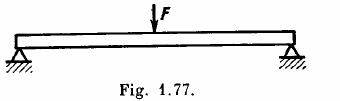Solution:

1. One can think of it as analogous to the previous case but with a beam of length l/2 loaded upward by a force F/2.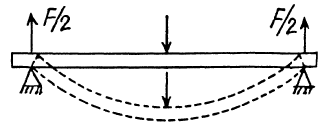Thus λ = Fl3/48 EI

On using the last result of the previous problem.

14. The thickness of a rectangular steel girder equals h. Using the equation of Problem 12, find the deflection λ caused by the weight of the girder in two cases:

1. (a) one end of the girder is embedded into a wall with the length of the protruding section being equal to l (Fig. 1.78a);

2. (b) the girder of length 2l rests freely on two supports (Fig. 1.78b).

Solution:

1. (a) In this case N(x) = ½ ρgbh(l-x)2 where b = width of the girder.

Also I = bh3/12. Then

(Ebh2/12)d2y/dx2 = (ρgbh/2)(l2 - 2lx + x2)

Integrating dy/dx = (6ρg/Eh2)(l2x - lx2 + xx3/3)

Using dy/dx for x = 0. Again integrating

y = (6ρg/Eh2)(l2x2/2 - lx3/3 + x4/12)

Thus λ = (6ρgl4/Eh2)(½ - ⅓ + 1/12)

= (6ρgl4/Eh2)(3/12)

= 3ρgl4/2Eh2

(b) As before, EI d2y/dx = N(x) where N(x) is the bending moment due to section PB. This bending moment is clearly

$N =\int_{x}^{2l}wd\xi (\xi -x)-wl(2l-x)$

= w(2l2 - 2xl + x2/2) - wl(2l - x)

= w(x2/2 - xl)

(here w = ρgbh is weight of the beam per unit length)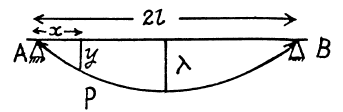Now integrating, EI dy/dx = w(x3/6 - x2l/2) + c0

Or since dy/dx = 0, for x = l, c0 = wl3/3

Integrating again, EIy = w(x4/24 - x3l/6) + wl3x/3 + c1

As y = 0 for x = 0, c1 = 0. From this we find

λ = y(x= l) = 5wl4/24EI

= 5ρgl4/2Eh2

15. A steel plate of thickness h has the shape of a square whose side equals l, with h << l. The plate is rigidly fixed to a vertical axle OO which is rotated with a constant angular acceleration β (Fig. 1.79). Find the deflection λ, assuming sagging to be small.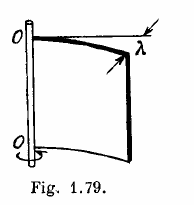Solution:

1. The deflection of the plate can be noticed by going to a co rotating frame. In this frame each element of the plate experiences a pseudo force proportional to its mass. These forces have a moment which constitutes the bending moment of the problem. To calculate this moment we note that the acceleration of an element at a distance ξ from the axis = a = ξ β and the moment of the forces exerted by the section between x and l is

$N =\rho lh \beta \int_{x}^{l}\xi ^{2}d\xi$

= ⅓ ρlhβ(l3 - x3)

From the fundamental equation

EI d2y/dx2 = ⅓ ρlhβ(l3 - x3)

The moment of inertia I = $\int_{\frac{-h}{2}}^{\frac{+h}{2}}z^{2}l\: dz$

= lh3/12

Note that the neutral surface (i.e. the surface which contains lines which are neither stretched nor compressed) is a vertical plane here and z is perpendicular to it.

d2y/dx2 = (4ρβ/Eh2)(l3 - x3)

Integrating

dy/dx = (4ρβ/Eh2)(l3x - x4/4) + c1

Since dy/dx = 0, for x = 0, c1 = 0. Integrating again,

y = (4ρβ/Eh2)(l3x2/2 - x5/20) + c2

c2 = 0 because y = 0 for x = 0

Thus λ = y(x= l) = 9ρβl5/5Eh2

16. Determine the relationship between the torque N and the torsion angle ϕ for

1. a) the tube whose wall thickness r is considerably less than the tube radius;

2. (b) for the solid rod of circular cross section. Their length l, radius r, and shear modulus G are supposed to be known.

Solution:

1. (a) Consider a hollow cylinder of length l, outer radius r + ∆r inner radius r, fixed at one end and twisted at the other by means of a couple of moment N. The angular displacement ϕ, at a distance l from the fixed end, is proportional to both l and N. Consider an element of length dx at the twisted end. It is moved by an angle ϕ as shown. A vertical section is also shown and the twisting of the parallelepiped of length l and area ∆r dx under the action of the twisting couple can be discussed by elementary means. If f is the tangential force generated then shearing stress is f/Δr dx and this must equal

Gθ = Grϕ/l, since θ = rϕ/l

Hence f = G∆r dx rϕ/l

The force f has moment fr about the axis and so the total moment is

N = G∆r (ϕ/l)r2∫ dx = 2πr3∆rϕG/l

(b) For a solid cylinder we must integrate over r. Thus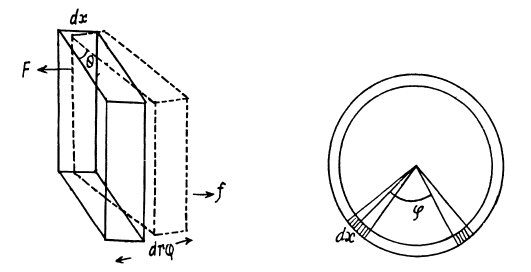$N=\int_{0}^{r}\frac{2\pi r^{3}\: dr\phi G}{l}$

= πr4Gϕ/2l

17. Calculate the torque N twisting a steel tube of length l = 3.0 m through an angle φ = 2.00 about its axis, if the inside and outside diameters of the tube are equal to d1 = 30 mm and d2 = 50 mm

Solution:

1. Clearly $N=\int_{\frac{d_{1}}{2}}^{\frac{d_{2}}{2}}\frac{2\pi r^{3}dr\varphi G}{l}$

= (π/32l)Gφ (d24 - d14)

Using G = 81GPa

= 8.1×1010 N/m2

d2 = 5×10-2 m

d1 = 3×10-2 m

φ = 2.00 = π/90 radian, l = 3m

N = (π×8.1×π(625-81)/32×3×90 )×102Nm

= 0.5033×103Nm

≈ 0.5 kNm

18. Find the maximum power which can be transmitted by means of a steel shaft rotating about its axis with an angular velocity ω = 120 rad/s, if its length l = 200 cm, radius r = 1.50 cm, and the permissible torsion angle φ = 2.50.

Solution:

1. The maximum power that can be transmitted by means of a shaft rotating about its axis is clearly Nω where N is the moment of the couple producing the maximum permissible torsion, φ. Thus

P = πr4Gφω/2l

= 16.9 kw

19. A uniform ring of mass m, with the outside radius r2, is fitted tightly on a shaft of radius r1. The shaft is rotated about its axis with a constant angular acceleration β. Find the moment of elastic forces in the ring as a function of the distance r from the rotation axis.

Solution:

1. Consider an elementary ring of width dr at a distance r from the axis. The part outside exerts a couple N + (dN/dr)dr on this ring while the part inside exerts a couple N in the opposite direction.

We have for equilibrium

(dN/dr)dr = -dlβ

where dl is the moment of inertia o f the elementary ring, β is the angular acceleration and minus sign is needed because the couple N (r) decreases, with distance vanishing at the outer radius, N (r2) = 0. Now

dI = (m/π(r22 - r12))2πr dr r2

Thus dN = 2mβ/(r22 - r12) r3dr

Or N = (½ mβ/(r22-r12))(r24 - r4), on integration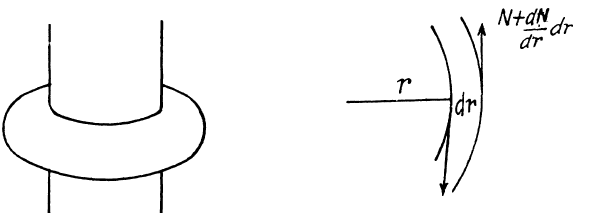20. Find the elastic deformation energy of a steel rod of mass m = 3.1 kg stretched to a tensile strain ε = 1.0×10-3

Solution:

1. We assume that the deformation is wholly due to external load, neglecting the effect of the weight of the rod (see next problem). Then a well known formula says, elastic energy per unit volume = ½ stress × strain

= ½ σ ε

This gives ½ m/ρ)Eε2 ≈ 0.04 kJ for the total deformation energy.